# NCERT Solutions for Class 10 Maths Chapter 15 Probability

NCERT Solutions Class 10 Maths Chapter 15 – Probability resource was curated by the GFG team to help students in answering questions and problems related to probability and its applications. All of the exercises in this chapter’s NCERT textbook exercise book are covered in the NCERT Solutions for Class 10 Maths.

Probability is the last chapter of the syllabus. The likelihood that an event will occur, basic event notions, the complement of events, and the theoretical approach to probability are all covered in this chapter. The chapter then goes on to describe the distinction between experimental probability and theoretical probability.

These Solutions cover all four exercises of the NCERT Class 10 Maths Chapter 15, which are as follows:

Class 10 Maths NCERT Solutions Chapter 15 Exercises

• NCERT Maths Solutions Class 10 Exercise 15.1 Set 1Set 2 – 25 Questions (22 Short Answers, 3 Long Answers)

## Probability: Exercise 15.1

Theorem 1:

The theoretical probability (also called classical probability) of an event E, written as P(E), is defined as

P(E) =### Question 1. Complete the following statements:

(i) Probability of an event E + Probability of the event ‘not E’ =_________________ .

(ii) The probability of an event that cannot happen is __________. Such an event is called __________________ .

(iii) The probability of an event that is certain to happen is ______________. Such an event _______________ is called .

(iv) The sum of the probabilities of all the elementary events of an experiment is ______________________.

(v) The probability of an event is greater than or equal to _______________and less than or _________________ equal to .

Solution:

(i) 1

(ii) 0, Impossible Event

(iii) 1, Sure Event

(iv) 1

(v) 0, 1

### Question 2. Which of the following experiments have equally likely outcomes? Explain.

(i) A driver attempts to start a car. The car starts or does not start.

(ii) A player attempts to shoot a basketball. She/he shoots or misses the shot.

(iv) A baby is born. It is a boy or a girl.

Solution:

(i) This statement does not have equally likely outcomes as the car may or may not start depending upon various internal and external factors like fuel, path etc.

(ii) This statement does not have equally likely outcomes as the player may shoot or miss the shot depending upon various factors.

(iii) This statement has equally likely outcomes as it is known that the solution is either right or wrong, both have equal chance to happen.

(iv) This statement also has equally likely outcomes as it is known that the newly born baby can either be a boy or a girl, both have equal chance to happen.

### Question 3. Why is tossing a coin considered to be a fair way of deciding which team should get the ball at the beginning of a football game?

Solution:

Tossing of a coin is a fair way of deciding because they have equally likely outcomes where the number of possible outcomes are only 2 i.e. either head or tail. Tossing is considered to be completely unbiased and is unpredictable .

### Question 4. Which of the following cannot be the probability of an event?

(A) 2/3

(B) -1.5

(C) 15%

(D) 0.7

Solution:

As the probability of any event (E) always lies between 0 and 1

i.e. 0 ≤ P(E) ≤ 1

(i) 2/3 can be the probability of an event.

(ii) -1.5 cannot be the probability of an event.

(iii) 15% can be the probability of an event.

(iv) 0.7 can be the probability of an event.

### Question 5. If P(E) = 0.05, what is the probability of ‘not E’?

Solution:

As, P(E) + P(not E) = 1

P(Not E) = 1 – P(E) (Where the event ‘not E’ is complement of the event E)

Here, P(E) = 0.05

Then, P(Not E) = 1 – 0.05

P(Not E) = 0.95

### Question 6. A bag contains lemon-flavored candies only. Malini takes out one candy without looking into the bag. What is the probability that she takes out

(i) an orange flavoured candy?

(ii) a lemon flavoured candy?

Solution:

(i) As we know that the bag contains lemon-flavored candies only.

Hence, the no. of orange flavored candies = 0

The probability of taking out orange flavored candies =…………………… (from Theorem 1)

= 0 (Impossible Event)

(ii) As there are only lemon flavored candies,

P(Lemon flavored candies) = 1 (Sure Event)

### Question 7. It is given that in a group of 3 students, the probability of 2 students not having the same birthday is 0.992. What is the probability that the 2 students have the same birthday?

Solution:

As, P(E) + P(not E) = 1

P(E) = 1 – P(Not E) (Where the event ‘not E’ is complement of the event E)

Here, P(Not E) = Probability of 2 students not having the same birthday = 0.992

Here, P(E) = Probability that the 2 students have the same birthday

Then, P(E) = 1 – 0.992

P(E) = 0.08

Hence, the probability that the 2 students have the same birthday is 0.008

### Question 8. A bag contains 3 red balls and 5 black balls. A ball is drawn at random from the bag. What is the probability that the ball drawn is

(i) red?

(ii) not red?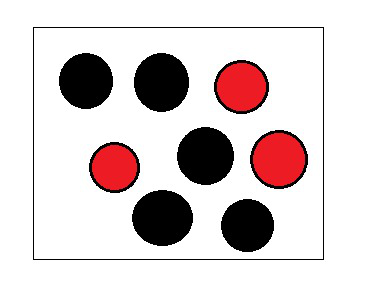Solution:

Number of Red balls = 3

Number of Black balls = 5

Total number of balls = 5+3 = 8

Hence, total number of possible outcomes = 8

(i) P(E) = Probability that the ball drawn is Red.

P(E) =……………..(From Theorem 1)

(ii) P(Not E) = Probability that the ball drawn is not Red

P(Not E) = 1 – P(E) (Where the event ‘not E’ is complement of the event E)

P(Not red) = 1-### Question 9. A box contains 5 red marbles, 8 white marbles, and 4 green marbles. One marble is taken out of the box at random. What is the probability that the marble taken out will be

(i) red?

(ii) white?

(iii) not green?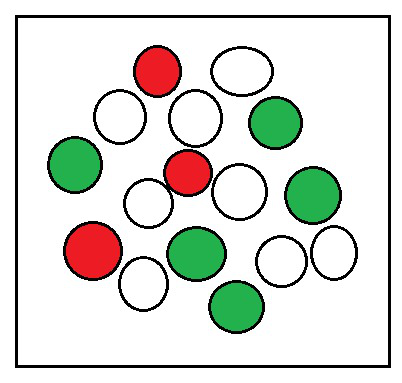Solution:

Number of Red balls = 5

Number of White balls = 8

Number of Green balls = 4

Total number of balls = 5+8+4 = 17

Hence, total number of possible outcomes = 17

(i) P(E) = Probability that the ball drawn is Red.

P(E) =…………….. (From Theorem 1)

(ii) P(E) = Probability that the ball drawn is White.

P(E) =…………….. (From Theorem 1)

(iii) Let P(E) = Probability that the ball drawn is not Green.

P(E) =………. (From Theorem 1)

P(Not E) = 1 – P(E) (Where the event ‘not E’ is complement of the event E)

P(Not Green) = 1-### Question 10. A piggy bank contains hundred 50p coins, fifty ₹ 1 coins, twenty ₹ 2 coins and ten ₹ 5 coins. If it is equally likely that one of the coins will fall out when the bank is turned upside down, what is the probability that the coin

(i) will be a 50 p coin?

(ii) will not be a ₹ 5 coin?

Solution:

Number of 50p coins = 100

Number of ₹1 coins = 50

Number of ₹2 coins = 20

Number of ₹5 coins = 10

Total number of coins = 100+50+20+10 = 180

Hence, total number of possible outcomes = 180

(i) P(E) = Probability that the coin will be a 50 p coin.

P(E) =…………….. (From Theorem 1)

(ii) Let P(E) = Probability that the coin will be a ₹5 coin.

P(E) =.……………. (From Theorem 1)

Then, P(Not E) = Probability that the coin will not be a ₹5 coin.

P(Not E) = 1 – P(E) (Where the event ‘not E’ is complement of the event E)

P(Not ₹5 coin) = 1-### Question 11. Gopi buys a fish from a shop for his aquarium. The shopkeeper takes out one fish at random from a tank containing 5 male fish and 8 female fish. What is the probability that the fish taken out is a male fish?

Solution:

Number of male fish = 5

Number of female fish = 8

Total number of fish in the tank = 5+8 = 13

Hence, total number of possible outcomes = 13

P(E) = Probability that the fish will be male.

P(E)………………. (From Theorem 1)

### Question 12. A game of chance consists of spinning an arrow that comes to rest pointing at one of the numbers 1, 2, 3, 4, 5, 6, 7, 8 (see Fig. 15.5 ), and these are equally likely outcomes. What is the probability that it will point at

(i) 8?

(ii) an odd number?

(iii) a number greater than 2?

(iv) a number less than 9?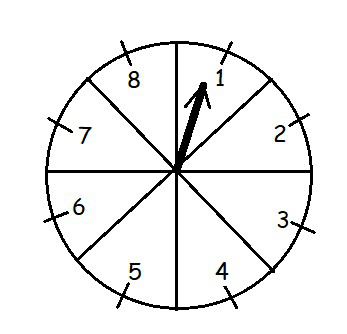Solution:

Here, total number of possible outcomes = 8

(i) P(E) = Probability that pointer will point at 8.

P(E)………………. (From Theorem 1)

(ii) P(E) = Probability that pointer will point at an odd number.

Odd number = {1,3,5,7}

P(E) =………………. (From Theorem 1)

(iii) P(E) = Probability that pointer will point at a number greater than 2.

Numbers greater than 2 = {3,4,5,6,7,8}

P(E) =………………. (From Theorem 1)

(iv) P(E) = Probability that pointer will point at a number less than 9.

Numbers less than 9 = {1,2,3,4,5,6,7,8}

P(E) == 1 ………………. (From Theorem 1)

### Question 13. A die is thrown once. Find the probability of getting:

(i) a prime number

(ii) a number lying between 2 and 6

(iii) an odd number.

Solution:

Here, total number of possible outcomes = 6

 1 2 3 4 5 6

(i) P(E) = Probability of getting a prime number.

Prime numbers = {2,3,5}

P(E) =………………. (From Theorem 1)

(ii) P(E) = Probability of getting a number between 2 and 6.

Numbers between 2 and 6 = {3,4,5}

P(E) =………………. (From Theorem 1)

(iii) P(E) = Probability of getting an odd number.

Odd numbers = {1,3,5}

P(E) =………………. (From Theorem 1)

### Question 14. One card is drawn from a well-shuffled deck of 52 cards. Find the probability of getting

(i) a king of red color

(ii) a face card

(iii) a red face card

(iv) the jack of hearts

(vi) the queen of diamonds

Solution:

Here, total number of possible outcomes = 52

(i) P(E) = Probability of getting a king of red colour.

Number king of red colour = 2

P(E) =………………. (From Theorem 1)

(ii) P(E) = Probability of getting a face card.

Number face cards = 12

P(E) =………………. (From Theorem 1)

(iii) P(E) = Probability of getting a red face card.

Number red face cards = 6

P(E) =………………. (From Theorem 1)

(iv) P(E) = Probability of getting the jack of hearts.

Number of jack of hearts = 1

P(E)………………. (From Theorem 1)

(v) P(E) = Probability of getting a queen of diamond.

Number of queen of diamonds = 1

P(E)………………. (From Theorem 1)

### Question 15. Five cards—the ten, jack, queen, king, and ace of diamonds, are well-shuffled with their face downwards. One card is then picked up at random.

(i) What is the probability that the card is the queen?

(ii) If the queen is drawn and put aside, what is the probability that the second card picked up is

(a) an ace?

(b) a queen?

Solution:

Here, total numbers of cards = 5

(i) P(E) = Probability of getting a queen.

P(E)………………. (From Theorem 1)

(ii) When the queen is put aside, then Here, total numbers of remaining cards = 4

(a) P(E) = Probability of getting an ace.

P(E)………………. (From Theorem 1)

(b) P(E) = Probability of getting a queen.

P(E) == 0 ………………. (From Theorem 1)

### Question 16. 12 defective pens are accidentally mixed with 132 good ones. It is not possible to just look at a pen and tell whether or not it is defective. One pen is taken out at random from this lot. Determine the probability that the pen taken out is a good one.

Solution:

Number of deflective pens = 12

Number of good pens = 132

Total number of pens = 12+132 = 144

Hence, total number of possible outcomes = 144

P(E) = Probability of getting a good pen.

P(E) =………………. (From Theorem 1)

### (ii) Suppose the not defective bulb drawn in and is not replaced. Now one bulb is drawn at random from the rest. What is the probability that this bulb is not defective?

Solution:

(i) Numbers of defective bulbs = 4

The total numbers of bulbs = 20

Hence, total number of possible outcomes = 20

P(E) = Probability of getting a defective bulb

P(E) =………………. (From Theorem 1)

(ii) As a non-defective bulb is drawn, then the total numbers of bulbs left are 19

Hence, the total numbers of outcomes = 19

Numbers of non-defective bulbs = 19-4 = 15

P(E) = Probability of getting a non-defective bulb

P(E)………………. (From Theorem 1)

### Question 18. A box contains 90 discs which are numbered from 1 to 90. If one disc is drawn at random from the box, find the probability that it bears

(i) a two-digit number

(ii) a perfect square number

(iii) a number divisible by 5.

Solution:

The total numbers of discs (outcomes) = 90

(i) Total number of discs having two-digit numbers = 81

(As 1 to 9 are single digit numbers)

P(E) = Probability of getting a two digit-number

P(E) =………………. (From Theorem 1)

(ii) Total numbers of perfect square number = 9

{1, 4, 9, 16, 25, 36, 49, 64 and 81}

P(E) = Probability of getting a perfect square

P(E) =………………. (From Theorem 1)

(iii) Total numbers which are divisible by 5 = 18

{5, 10, 15, 20, 25, 30, 35, 40, 45, 50, 55, 60, 65, 70, 75, 80, 85 and 90}

P(E) = Probability of getting a number divisible by 5.

P(E) =………………. (From Theorem 1)

### Question 19. A child has a die whose six faces show the letters as given below :

 A B C D E A

### The die is thrown once. What is the probability of getting

(i) A?

(ii) D?

Solution:

The total numbers of outcomes = 6

(i) P(E) = Probability of getting A

Total number of ‘A’ in dice = 2

P(E) =………………. (From Theorem 1)

(ii) P(E) = Probability of getting D

Total number of ‘D’ in dice = 1

P(E)………………. (From Theorem 1)

### Question 20. Suppose you drop a die at random on the rectangular region shown in Fig. 15.6. What is the probability that it will land inside the circle with diameter 1m?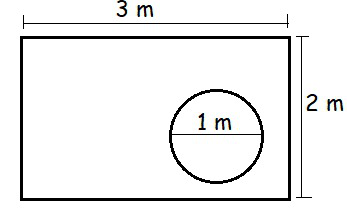Solution:

Here, the area of the rectangle is the possible outcome and,

The area of the circle will be the favourable outcome.

So, the area of the rectangle = (length × breadth) = (3×2) = 6 m2

and, the area of the circle = πr2 = π(½)2 = π/4 m2

P(E) = The probability that die will land inside the circle

P(E) =### Question 21. A lot consists of 144 ball pens of which 20 are defective and the others are good. Nuri will buy a pen if it is good, but will not buy if it is defective. The shopkeeper draws one pen at random and gives it to her. What is the probability that

(ii) She will not buy it?

Solution:

Number of defective pens = 20

Total number of pens (outcomes)= 144

(i) P(E) = The probability that she will buy =  The probability of getting a good pens

Number of good pens = 144-20 = 124

P(E) =………………. (From Theorem 1)

(ii) P(E) = The probability that she will not buy =  The probability of getting a defective pens

P(E) =………………. (From Theorem 1)

### Question 22. Refer to Example 13.

(i) Complete the following table: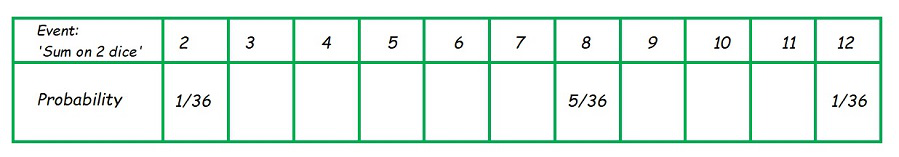(ii) A student argues that ‘there are 11 possible outcomes 2, 3, 4, 5, 6, 7, 8, 9, 10, 11 and 12. Therefore, each of them has a probability 1/11. Do you agree with this argument? Justify your answer.

Solution:

When two dices are thrown, then the total outcomes = 36

 (1,1) (1,2) (1,3) (1,4) (1,5) (1,6) (2,1) (2,2) (2,3) (2,4) (2,5) (2,6) (3,1) (3,2) (3,3) (3,4) (3,5) (3,6) (4,1) (4,2) (4,3) (4,4) (4,5) (4,6) (5,1) (5,2) (5,3) (5,4) (5,5) (5,6) (6,1) (6,2) (6,3) (6,4) (6,5) (6,6)

(i) P(E) = Probability of having sum as 2(Given)

Sample space is (1,1)

P(E) = Probability of having sum as 3

Sample space are (1,2) and (2,1)

P(E) =P(E) = Probability of having sum as 4

Sample space are (1,3), (3,1), and (2,2)

P(E) =P(E) = Probability of having sum as 5

Sample space are (1,4), (4,1), (2,3), and (3,2)

P(E) =P(E) = Probability of having sum as 6

Sample space are (1,5), (5,1), (2,4), (4,2), and (3,3)

P(E)P(E) = Probability of having sum as 7

Sample space are (1,6), (6,1), (5,2), (2,5), (4,3), and (3,4)

P(E) =P(E) = Probability of having sum as 8

Sample space are (2,6), (6,2), (3,5), (5,3), and (4,4)

P(E)P(E) = Probability of having sum as 9

Sample space are (3,6), (6,3), (4,5), and (5,4)

P(E) =P(E) = Probability of having sum as 10

Sample space are (4,6), (6,4), and (5,5)

P(E) =P(E) = Probability of having sum as 11

Sample space are (5,6), and (6,5)

P(E) =P(E) = Probability of having sum as 12

Sample space is (6,6)

P(E)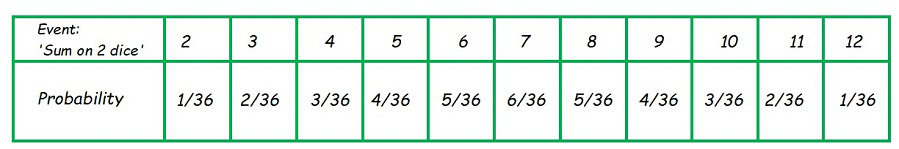(ii) The argument is not correct as it contradicts the verified solution in (i) that the number of all possible outcomes is 36 and not 11.

### Question 23. A game consists of tossing a one rupee coin 3 times and noting its outcome each time. Hanif wins if all the tosses give the same result i.e., three heads or three tails, and loses otherwise. Calculate the probability that Hanif will lose the game.

Solution:

The total number of outcomes = 8, as follows

 HHH HHT HTT HTH THT THH TTH TTT

Total outcomes in which Hanif will lose the game = 6

 HHT HTT HTH THT THH TTH

P(E) = The probability that he will lose

P(E) =### Question 24. A die is thrown twice. What is the probability that

(i) 5 will not come up either time?

(ii) 5 will come up at least once?

[Hint: Throwing a die twice and throwing two dice simultaneously are treated as the same experiment]

Solution:

When two dices are thrown, then the total outcomes = 36

 (1,1) (1,2) (1,3) (1,4) (1,5) (1,6) (2,1) (2,2) (2,3) (2,4) (2,5) (2,6) (3,1) (3,2) (3,3) (3,4) (3,5) (3,6) (4,1) (4,2) (4,3) (4,4) (4,5) (4,6) (5,1) (5,2) (5,3) (5,4) (5,5) (5,6) (6,1) (6,2) (6,3) (6,4) (6,5) (6,6)

(i) P(E) = Probability that 5 will not come up either time

5 will not come up either time = (36-11) = 25

P(E)………………..(From Theorem 1)

(ii) P(E) = Probability that 5 will not come up at least once

P(E)…………………(From Theorem 1)

### Question 25. Which of the following arguments are correct and which are not correct? Give reasons for your answer.

(i) If two coins are tossed simultaneously there are three possible outcomes—two heads, two tails, or one of each. Therefore, for each of these outcomes, the probability is 1/3.

(ii) If a die is thrown, there are two possible outcomes—an odd number or an even number. Therefore, the probability of getting an odd number is 1/2.

Solution:

(i) The total number of outcomes = 4, as follows

 HH HT TH TT

So, P (getting two heads) = 1/4

P (getting two tails) = 1/4

and, P (getting one of the each) =Hence, this statement is INCORRECT.

(ii) Total outcomes = 6

 1 2 3 4 5 6

P(Odd) = Probability of getting an odd number.

Odd numbers = {1,3,5}

P(Odd) =………………(I). (From Theorem 1)

From (I) we conclude that,

The probability of getting an odd number is 1/2.

Hence, this statement is CORRECT.

## Probability: Exercise 15.2

### Question 1. Two customers Shyam and Ekta are visiting a particular shop in the same week (Tuesday to Saturday). Each is equally likely to visit the shop on any day as on another day. What is the probability that both will visit the shop on (i) the same day? (ii) consecutive days? (iii) different days?

Solution:

Total number of days = 5 (Tuesday, Wednesday, Thursday, Friday, Saturday)

Shyam can visit the shop in any of the 5 days.

Ekta can visit the shop in any of the 5 days.

So, total number of outcomes  = (5 × 5) = 25

(i) Probability that both will visit the shop on the same day P(E);

They both will visit the shop either on Tuesday or Wednesday or Thursday or Friday or Saturday.

P(E) = 5 / 25  = 1 / 5

(ii) Probability that both will visit the shop on consecutive days P(E);

Consecutive means the following day.

So, they may visit the shop in following days; (T, W), (W, TH), (TH, F), (F, S),  (W, T), (TH, W),  (F, TH),  (S, F)

They can visit the shop in 8 ways.

P(E) = 8 / 25

(iii) Probability that both will visit the shop on different days P(E);

We know that, P(E) + P(Ē) = 1

i.e.. Probability of visiting the shop in same day + Probability  of visiting the shop in  different days = 1

Probability of visiting the shop in same day P(E)  = 1/ 5

Therefore, Probability that both will visit the shop on different days P(Ē) = 1 – P(E)

= 1 – (1) / 5

= 4 / 5

### Question 2. A die is numbered in such a way that its faces show the numbers 1, 2, 2, 3, 3, 6. It is thrown two times and the total score in two throws is noted. Complete the following table which gives a few values of the total score on the two throws:

 + 1 2 2 3 3 6 1 2 3 3 4 4 7 2 3 4 4 5 5 8 2 5 3 3 5 9 6 7 8 8 9 9 12

### (i) even? (ii) 6? (iii) at least 6?

Solution:

The given table can be completed as below.

 + 1 2 2 3 3 6 1 2 3 3 4 4 7 2 3 4 4 5 5 8 2 3 4 4 5 5 8 3 4 5 5 6 6 9 3 4 5 5 6 6 9 6 7 8 8 9 9 12

Two dice have been thrown. So the total number of outcomes = (6 × 6) = 36

(i) Probability that the total score is even P(E);

From, the above table, below are the throws in which the total score is even; (1, 1), (1, 3), (1, 3), (2, 2), (2, 2), (6, 2), (2, 2), (2, 2), (6, 2), (1, 3), (3, 3), (3, 3), (1, 3), (3, 3), (3, 3), (2, 6), (2, 6), (6, 6)

Total number of throws, in which the total score is even = 18

P(E) = 18 / 36 = 1/ 2

(ii) Probability that the total score is 6 P(E);

Events that produce total score of 6 = (3, 3), (3, 3), (3, 3), (3, 3)

Total number of throws, in which the total score is 6 = 4

P(E) = 4 / 36 = 1/ 9

(iii) Probability that the total score is atleast 6 P(E);

At least means that greater than or equal to 6 (>=).

Events that produce total score of 6 = (1, 6), (2, 6), (2, 6), (3, 3), (3, 3), (3, 6), (3, 3), (3, 3), (3, 6), (6, 1), (6, 2), (6, 2), (6, 3), (6, 3), (6, 6)

Total number of throws, in which the total score is atleast 6 = 15

P(E) = 15 / 36 = 5 / 12

### Question 3. A bag contains 5 red balls and some blue balls. If the probability of drawing a blue ball is double that of a red ball, determine the number of blue balls in the bag?

Solution:

Total number of red balls = 5

Total number of blue balls = ?

Let, the total number of blue balls be = x

Then the total number of balls in the bag (both red and blue) = (5 + x)

Probability of drawing a red ball P(E) = 5 / (5 + x)

Probability of drawing a blue ball P(E) = x / (5 + x)

Given that, probability of drawing a blue ball is double that of a red ball;

(x / (5 + x)) = 2(5 / (5 + x))

=> x / (5 + x) =  10 / (5 + x)

=> 5x + x2 = 50 + 10x

=> x2 + 5x – 10x – 50 = 0

=> x2 – 5x + 50 = 0

=> (× – 10) (x + 5) = 0

Therefore,

x – 10 = 0 , x = 10

x + 5 = 0 , x = -5

As, the probability cannot be negative (probability lies between  0 to 1);

the x will be 10.

Total number of blue balls in the bag = 10.

### Question 4. A box contains 12 balls out of which x are black. If one ball is drawn at random from the box, what is the probability that it will be a black ball? If 6 more black balls are put in the box, the probability of drawing a black ball is now double what it was before. Find x?

Solution:

The box contains = 12 balls

Number of black balls = x

Other balls = (12 – x)

Probability that the ball drawn will be a black ball P(E);

P(E) = x / 12

Now,  total number of balls  = 12 + 6 = 18

total black balls in the bag = (6 + x)

Probability of drawing a black ball is now double of what it was before

Probability of drawing a black ball = (6 + x) / 18

=> (6 + x) / 18 = 2 (x / 12)

=> 12 ( 6 +  x )  = 18 ( 2x )

=> 2 ( 6 + x ) = 3 ( 2x )

=> 12 + 2x = 6x

=> 12  = 6x – 2x

=> 12 = 4x

=> 3 = x

Number of black balls = 3

### Question 5. A jar contains 24 marbles, some are green and others are blue. If a marble is drawn at random from the jar, the probability that it is green is 2/3⋅ Find the number of blue balls in the jar.

Solution:

The jar contains – 24 marbles

Let the number of green marbles be = x

Probability of drawing a green marble;

P(E) = 2 / 3

i.e. x / 24 = 2 / 3

=> 3x  = 48

=> x = 16

Therefore, the number of blue marbles in the jar = 24 – 16  =  8

### Important Points to Remember:

• These NCERT solutions are developed by the GfG team, with a focus on students’ benefit.
• These solutions are entirely accurate and can be used by students to prepare for their board exams.
• Each solution is presented in a step-by-step format with comprehensive explanations of the intermediate steps.

## FAQs on NCERT Solutions for Class 10 Maths Chapter 15 Probability

### Q1: Why is it important to learn probability?

It is important to learn probability because it helps in understanding uncertainty and making informed decisions based on likelihood. One of the key reasons to learn probability is its real-world applications. By understanding probability, students can analyze data, model uncertain events, and make predictions. This knowledge is essential for fields like weather forecasting, sports predictions, and risk assessment in insurance. It is utilized in a variety of disciplines, including data science, engineering, finance, and statistics.

### Q2: What topics are covered in NCERT Solutions for Class 10 Maths Chapter 15 – Probability?

NCERT Solutions for Class 10 Maths Chapter 15 – Probability covers topics such

### Q3: How can NCERT Solutions for Class 10 Maths Chapter 15 – Probability help me?

NCERT Solutions for Class 10 Maths Chapter 15 – Probability can help you solve the NCERT exercise without any limitations. If you are stuck on a problem you can find its solution in these solutions and free yourself from the frustration of being stuck on some question.

### Q4: How many exercises are there in Class 10 Maths Chapter 15 – Probability?

There are 2 exercises in the Class 10 Maths Chapter 15 – Probability which covers all the important topics and sub-topics.